Tip / Sign in to post questions, reply, level up, and achieve exciting badges. Know more

# Radar sensor Forum Discussions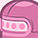Level 4Level 4

# the theoretical angular resolution of the BGT60TR13C

Hi,
Please let me know the theoretical angular resolution of the BGT60TR13C?
I think that it is depended on the distance between the Rx ANT. Is it correct?

Best regards,
H.N

1 SolutionModeratorModerator

# Re: the theoretical angular resolution of the BGT60TR13C

Hi @h_nakamura ,

Yeah, the θres given in above diagram does represent the angular resolution, cause both target A and target C are at same radial distance from the RADAR, but at different angle.

The BGT60TR13C does not have angular resolution, due to hardware limitations.

Thanks and regards,
Yashraj

11 Replies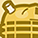Level 3Level 3

# Re: the theoretical angular resolution of the BGT60TR13C

How about your idea? In my opinion:

case 1:
Assume: lambda = 0.005 (60GHz -center freq),
- d = lambda/2 (fixed in bgt60tr13c)
- numRX = 2 ( Azimuth, fixed in bgt60tr13c)
if you using a very basic algorithm to calculate DoA that we call Angle-FFT, the angular resolution depends on this formula:
theta(res) = lambda/ (numRX * d * cos(theta))
So, from this formula, the angular resolution depends on (1) the number of Rx, and (2) DoA. For example, numRx =2 & target's position at 0 Deg then we get the res = 1. The accuracy of your calculations is good at theta = 0 Deg and reduces when theta goes to 90 Deg

it depends on your computing cost. Suppose if you use DAS || Capon || MUSIC, you can compute the weight value for your angle step( that step depends on you). For example, you can define a step like this 'angles(-90:1:90)' then a computing loop will follow that setting.Level 4Level 4

# Re: the theoretical angular resolution of the BGT60TR13C

Hi, Sorry for my late response.
I studied it and I think that the theoretical angular resolution depends on the distance(d) between Rx1/Rx3 and Rx2/Rx3.
It of the BGT60TR13C is 2.5mm by the datasheet.
Therefore,
θres>λ/(Ndcosθ)=λ/Nd=2/N=1°, ∵λ=5mm, d=2.5mm, N=2, d=λ/2
Is the theoretical angular resolution of the BGT60TR13C is 1°?
Is it correct?
Best regards,
N.NLevel 3Level 3

# Re: the theoretical angular resolution of the BGT60TR13C

Regarding angular resolution, there are many aspects. as I mentioned there are design rules for the distance between Rx and it is almost always used with a ratio of lambda/2. Since btg60tr13c has a fixed d and two antennas (Az||Ele), the angular resolution for an object only fluctuates according to its angle of incidence (directivity of an antenna & non-linear problem of sin function). However, be careful if you think a good resolution will help you identify multiple targets over the same distance; I can say that is not enough. To do that, you need a suitable "multi-input multi-output" (MIMO) system, which means the most important thing is the number of Tx||RX antennas you have. I hope this video helps you.Level 4Level 4

# Re: the theoretical angular resolution of the BGT60TR13C

Hi,
I understood the theory but do you know how much the angular resolution of the BTG60TR13C when I used the segmentation application of the GUI?
Best regards,
H.NModeratorModerator

# Re: the theoretical angular resolution of the BGT60TR13C

Hi @h_nakamura ,

There is no angular resolution for the BGT60TR13C radar. Thus, two targets in the same RangeDoppler bin can't be distinguished.

Thanks and regards,
YashrajLevel 4Level 4

# Re: the theoretical angular resolution of the BGT60TR13C

Hi Yashraj,
Because if the parameters are below then the Distance resolution at 4.8 is 0.15m, is it possible to say that the angular resolution is appx 1.7 degree?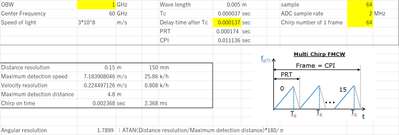Best regards,
H.NModeratorModerator

# Re: the theoretical angular resolution of the BGT60TR13C

Hi @h_nakamura ,

Range Resolution R­res :It is the minimum difference in radial distance from the RADAR which can be distinguished by RADAR. In the figure, if the RADAR can distinguish between the Target A and Target B, they must have difference in radial distance greater than or equal to range resolution R­res.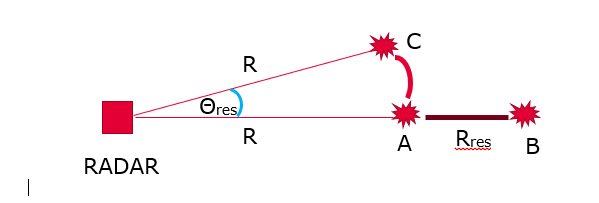Angular Resolution Θres : If the two targets A and C which are radially at equal distance from the RADAR, can be distinguished by the RADAR. Then, the RADAR has angular resolution equal to the angular difference between the two targets.

But from the formula you are using that is :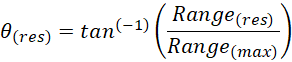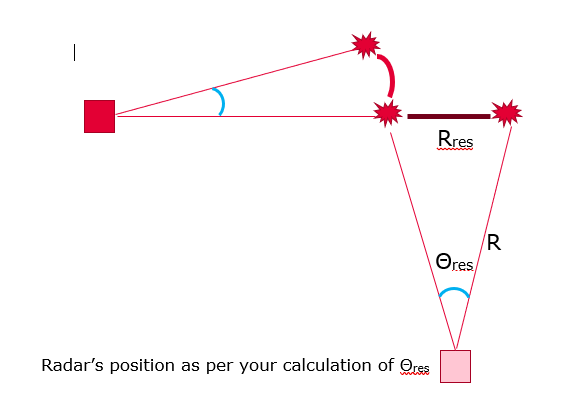In the figure you can see that it gives false perspective on the RADAR's position, which is inaccurate. Thus to summarize the formula you used can't give you angular resolution. As @85258, did mention in their reply that we require a MIMO device for angular resolution. For BGT60TR13C we can't deduce angular resolution, cause of its hardware limitations. You can use a MIMO device such as BGT60ATR24C if you want angular resolution.

Thanks and best regards
YashrajLevel 4Level 4

# Re: the theoretical angular resolution of the BGT60TR13C

Hi Yashraj,
Thanks for your explanation.
I'm litle bit confuse. If it is possible to distinguish A and B then the θres at this time is what is it?
Best regars,
H.NModeratorModerator

# Re: the theoretical angular resolution of the BGT60TR13C

Hi @h_nakamura ,

The difference between Target A and Target B with reference to RADAR, is that they are radially at different distances, so θres doesn't play a role there.
θres is the parameter that distinguishes between the two targets, at same radial distance from the RADAR.

Thanks and regards,
YashrajLevel 4Level 4

# Re: the theoretical angular resolution of the BGT60TR13C

I thought the angular resolution to be θres under the following conditions. By the way, what is the minimum θres of BGT60TR13C？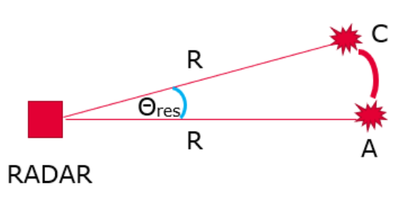ModeratorModerator

# Re: the theoretical angular resolution of the BGT60TR13C